# Java Program to find smallest of three numbers using ternary operator

In this tutorial you will learn about the Java Program to find smallest of three numbers using ternary operator and its application with practical example.

In this tutorial, we will learn to create Java Program to find smallest  of three numbers using ternary operator using Java programming.

## Prerequisites

Before starting with this tutorial we assume that you are best aware of the following Java programming topics:

• Java Operators.
• Basic Input and Output function in Java.
• Class and Object in Java.
• Basic Java programming.
• If-else statements in Java.
• For loop in Java.

### Ternary Operator Syntax :

The use of a ternary operator in our program decreases the number of lines of code and increases performance .

### Syntax of ternary Operator.

variable = condition ? expression1 : expression2 ;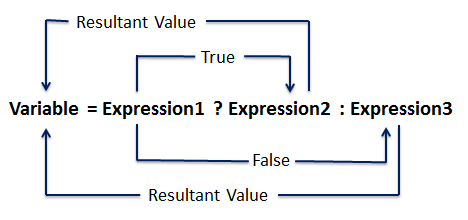## Java Program to find smallest of three numbers using ternary operator

In this program we would find smallest of three numbers using ternary operator . First of we would take three values from user and find the find smallest of three numbers using ternary operator. Let’s have a look at the code.

## Output

Smallest among three.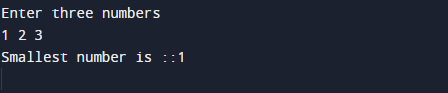In the above program, we have first declared and initialized a set variables required in the program.

• a,b and c = it will hold entered numbers.
• result=  it will hold result in it.
• temp = it will hold temporary answer.

After that we take three numbers from user and find smallest among three using ternary operator.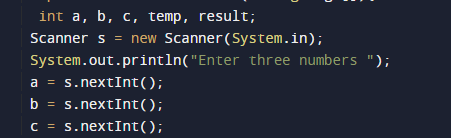after taking values from users we use ternary operator to calculate smallest among three

Boolean result= Condition ? true value : false value; it returns the Boolean value(true/false).
Logic behind the above expression is explained.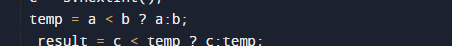And finally we print the result as shown in the image below.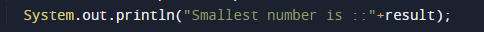So here the example  of finding smallest among three using ternary operator.

In this tutorial we have learn about the Java Program to find smallest of three numbers using ternary operator and its application with practical example. I hope you will like this tutorial.